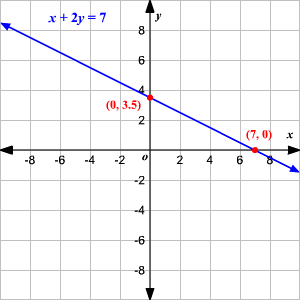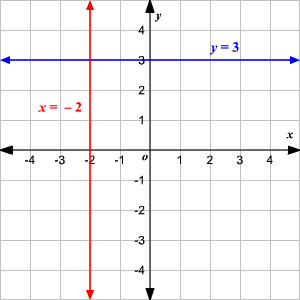# Graphing Linear Equations

The graph of a linear equation in two variables is a line (that's why they call it linear ).

If you know an equation is linear, you can graph it by finding any two solutions

$\left({x}_{1},{y}_{1}\right)$ and $\left({x}_{2},{y}_{2}\right)$ ,

plotting these two points, and drawing the line connecting them.

Example 1:

Graph the equation $x+2y=7$ .

You can find two solutions, corresponding to the $x$ -intercepts and $y$ -intercepts of the graph, by setting first $x=0$ and then $y=0$ .

When $x=0$ , we get:

$\begin{array}{l}0+2y=7\\ y=3.5\end{array}$

When $y=0$ , we get:

$\begin{array}{l}x+2\left(0\right)=7\\ x=7\end{array}$

So the two points are $\left(0,3.5\right)$ and $\left(7,0\right)$ .

Plot these two points and draw the line connecting them.If the equation is in slope-intercept form or point-slope form , you can also use the slope to help you graph.

Example 2:

Graph the line $y=3x+1$ .

From the equation, we know that the $y$ -intercept is $1$ , the point $\left(0,1\right)$ and the slope is $3$ .  Graph the point $\left(0,1\right)$ and from there go up $3$ units and to the right $1$ unit and graph a second point.  Draw the line that contains both points.Horizontal and vertical lines have extra simple equations.

Example 3:

Horizontal line: $y=3$

Vertical line: $x=-2$